### IMO Shortlist 2015 problem G6

Kvaliteta:
Avg: 0,0
Težina:
Avg: 8,0

Let$ABC$ be an acute triangle with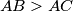$AB > AC$. Let$\Gamma$ be its cirumcircle,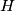$H$ its orthocenter, and$F$ the foot of the altitude from$A$. Let$M$ be the midpoint of$BC$. Let$Q$ be the point on$\Gamma$ such that$\angle HQA = 90^{\circ}$ and let$K$ be the point on$\Gamma$ such that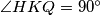$\angle HKQ = 90^{\circ}$. Assume that the points$A$,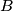$B$,$C$,$K$ and$Q$ are all different and lie on$\Gamma$ in this order.

Prove that the circumcircles of triangles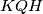$KQH$ and$FKM$ are tangent to each other.

(Ukraine)

Izvor: https://www.imo-official.org/problems/IMO2015SL.pdf